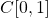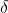# Chapter 22 of 24 - Compactness

The first section here was a stern-sounding "Two Important Theorems". It covered the boundedness theorem and the extreme value theorem, both I've covered before in previous pure maths modules, but this related them directly to metric spaces.

Second section decided to introduce compactness through the concept of sequential compactness, which essentially describes the "d-closed and bounded"-ness of a set. Though is inevitably difficult to learn quickly because of strange concepts involving sequences in metric spaces.

Third was "functions and sequential compactness", which was even more strange as it extended section two to also cover all functions in, and various hard-to-understand-theorems.

Section four was "compact metric spaces" and the notion of covers and sub covers. Far easier to understand, but depending on the set it can be tricky (for example) to find a finite sub cover from an infinite cover. With a bit of practise you get the trick though.

Section five was how sequential compactness and compactness are equivalent for metric spaces, and the last section covered unions and intersections of compact spaces. Again, these sections I sped through as section 5 was largely theorems, corollaries and lemmas, and section 6 was largely unexamined.